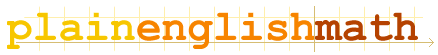You are here: MathematicsVectors → Vectors

# Vectors

Presumably, you've already read the page on the one-dimensionality of ordinary numbers (or scalars), in the Fundamentals section of this site. If not, you may wish to go back and browse that section before you continue reading this page.

Introduction
What is a vector?

The Cartesian coordinate system
Vectors don't just live on number lines, you know.

Other coordinate systems
There are many ways of writing down vectors.

Vector arithmetic
how to add, subtract and multiply vectors

Vector algebra
Just like scalar algebra. When you use a pronumeral to stand for a vector, it doesn't matter what coordinate system you use.